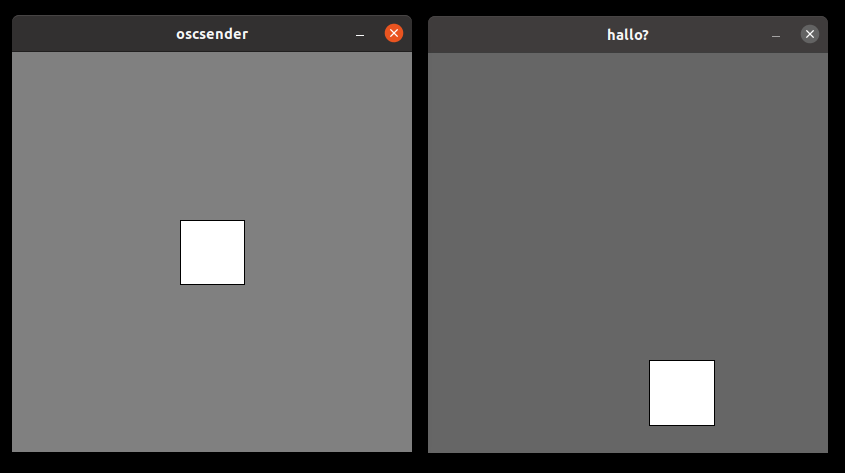## sketch experiment 7 - osc events

for my latest sketch experiment I implemented a osc event receiver that changes the size of a bouncing rectangle. The osc events are sent by a processing sketch.the osc part uses the cl-osc library. The receiver takes a int parameter and stores it in a global variable that is also used by the sketch to draw the rectangle.

``````(defvar si 10)
(defvar o)
(let ((s (socket-connect nil nil
:local-port port
:local-host #(127 0 0 1)
:protocol :datagram
:element-type '(unsigned-byte 8)))
(buffer (make-sequence '(vector (unsigned-byte 8)) 1024)))
(format t "Listening to localhost port ~A~%~%" port)
(unwind-protect
(loop do
(setf o (osc:decode-bundle buffer))
(setf si (car (rest o)))

(when s (socked-closed s)))))

(defsketch blub ())

(make-instance 'blub )

(defsketch blub ( (title "hallo?") (width 400) (height 400) (f 0) (dirx 2) (diry 1.3) (posx 0) (posy 0))
(incf f)
(setf posx (+ posx dirx))
(setf posy (+ posy diry))

(if (> posx (- width si)) (setf dirx (* dirx -1)))
(if (< posx 0) (setf dirx (* dirx -1)))
(if (> posy (- height si)) (setf diry (* diry -1)))
(if (< posy 0) (setf diry (* diry -1)))

(rect posx posy si si)
)

``````

this is the processing sketch I used to generate the osc messages. Each time the window is clicked, a new random size is generated and sent to the lisp sketch

``````import oscP5.*;
import netP5.*;
int f = 10;
OscP5 oscP5;

void setup() {
size(400,400);
oscP5 = new OscP5(this, 11111);
}

void draw() {
background(128);
translate(width/2,height/2);
rect(-f/2,-f/2,f, f);
}

void mousePressed() {
/* in the following different ways of creating osc messages are shown by example */
OscMessage myMessage = new OscMessage("/test");
f =   10 + (int)random(100);
println(f);